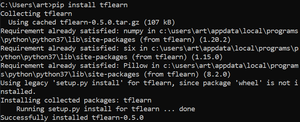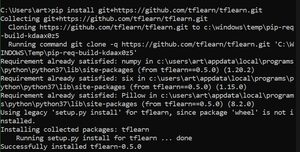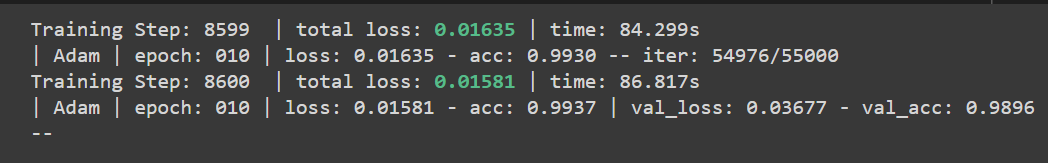Open in App
Not now

# TFLearn And Its Installation in Tensorflow

• Last Updated : 28 Nov, 2021

TFLearn can be described as a transparent and modular deep learning library created on top of the Tensorflow framework. The prime objective of TFLearn is to provide a higher-level API to Tensorflow so as to facilitate and accelerate experimentation, yet remaining fully compatible and transparent with it.

## Features

• TFLearn is easy to understand and is a user-friendly high-level API for building deep neural networks structure.
• Through built-in high compatibility of neural network layers, optimizers, regularizers, metrics, etc. it performs fast prototyping.
• TFLearn functions can be used independently as well since all functions are built upon tensors.
• With the use of powerful helper functions, any TensorFlow graph can be trained easily that accepts multiple inputs, outputs, and optimizers, etc.
• TFLearn can also be used to create magnificent graph visualizations with details about gradients, weights, activations, etc. effortlessly.
• Easy device placement for utilizing multiple CPU/GPU.

Many recent popular deep learning network architectures such as Convolutions, Residual networks, LSTM, PReLU, BatchNorm, Generative Networks, etc are supported by this high-level API.

Note: TFLearn v0.5 is only compatible with TensorFlow version 2.x

## Install TFLearn by executing this command:

For stable version:

`pip install tflearn`For state-of-art version:

`pip install git+https://github.com/tflearn/tflearn.git`## Example of tflearn:

Through the example given below application of TFLearn Regression is demonstrated.

## Python3

 `# Importing tflearn library ``import` `tflearn``from` `tflearn.layers.conv ``import` `conv_2d, max_pool_2d, input_data``from` `tflearn.layers.core ``import` `dropout, fully_connected``from` `tflearn.layers.estimator ``import` `regression``import` `tflearn.datasets.mnist as mnist`` ` `# Extracting MNIST dataset & dividing into ``# training and validation dataset``x_train,y_train,x_test,y_test ``=` `mnist.load_data(one_hot``=``True``)`` ` `# Reshaping dataset from (55000,784) to (55000,28,28,1) ``# using reshape``x_train ``=` `x_train.reshape([``-``1``, ``28``, ``28``, ``1``])``x_test ``=` `x_test.reshape([``-``1``, ``28``, ``28``, ``1``])`` ` `# Defining input shape (28,28,1) for network ``network ``=` `input_data(shape``=``[``None``, ``28``, ``28``, ``1``], name``=``'input'``)`` ` `# Defining conv_2d layer ``# Shape: [None,28,28,32]``network ``=` `conv_2d(network, ``32``, ``2``, activation``=``'relu'``)`` ` `# Defining max_pool_2d layer ``# Shape: [None,14,14,32]``network ``=` `max_pool_2d(network, ``2``)`` ` `# Defining conv_2d layer ``# Shape: [None,28,28,64]``network ``=` `conv_2d(network, ``64``, ``2``, activation``=``'relu'``)`` ` `# Defining max_pool_2d layer ``# Shape: [None,7,7,64]``network ``=` `max_pool_2d(network, ``2``)`` ` `# Defining fully connected layer ``# Shape: [None,1024]``network ``=` `fully_connected(network, ``512``, activation``=``'relu'``)`` ` `# Defining dropout layer ``# Shape: [None,1024]``network ``=` `dropout(network, ``0.3``)`` ` `# Defining fully connected layer``# Here 10 represents number of classes ``# Shape: [None,10]``network ``=` `fully_connected(network, ``10``, activation``=``'softmax'``)`` ` `# Defining regression layer``# Passing last fully connected layer as parameter, ``# adam as optimizer, ``# 0.001 as learning rate, categorical_crossentropy as loss ``# Shape: [None,10]``network ``=` `regression(network, optimizer``=``'adam'``, ``                     ``learning_rate``=``0.001``, ``                     ``loss``=``'categorical_crossentropy'``, ``                     ``name``=``'targets'``)`` ` `# Passing network made as parameter``model ``=` `tflearn.DNN(network)`` ` `# Fitting the model with training set:{x_train, y_train} ``# testing set:{x_test, y_test}``model.fit({``'input'``: x_test}, {``'targets'``: y_test}, ``          ``n_epoch``=``10``, ``          ``snapshot_step``=``500``, run_id``=``'mnist'``,``        ``validation_set``=``({``'input'``: x_test}, {``'targets'``: y_test}), ``        ``show_metric``=``True``)`

Output:Explanation:

In order to implement a classifier using tflearn, the first step is to import tflearn library and sub-modules like conv (for convolution layers), core (for dropout and fully connected layer), estimator (to apply linear or logistic regression), and datasets (to access datasets like MNIST, CIFAR10, etc.)

Using load_data, the MNIST dataset is extracted and divided into training and validation set with input x having a shape (samples,784). Now in order to use input x for training we reshape it from (samples,784) to (samples, 28,28,1) using .reshape(NewShape). After this, we define the same new shape for the network. Now for defining the network model we stack a few convolution2d layers and max_pooling2d layers together followed by stacked dropout and fully connected layers.

My Personal Notes arrow_drop_up